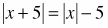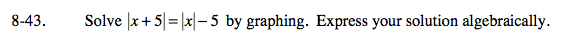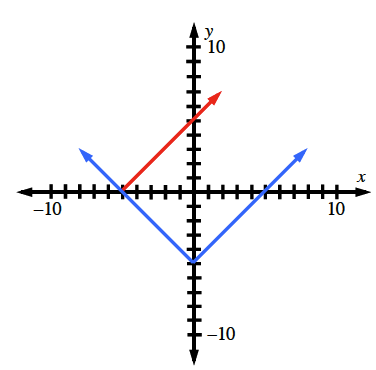Home > A2C > Chapter 8 > Lesson 8.1.3 > Problem8-43

8-43.

Solveby graphing. Express your solution algebraically. Homework Help ✎The solution is the value of x that makes both of these equations true. Where do the graphs overlap?

x ≤ −5